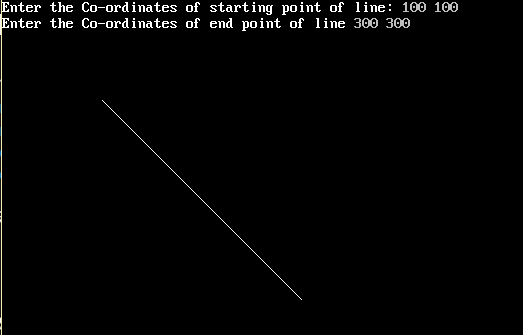×

Search anything:

# DDA (Digital Differential Analyzer) Line Drawing Algorithm

#### Algorithms computer graphics line drawing algorithmGet this book -> Problems on Array: For Interviews and Competitive Programming

Reading time: 15 minutes | Coding time: 10 minutes

Digital differential analyzer is a line drawing algorithm that is based on incremental method, which calculates all intermediate points over the interval between start and end points.### Algorithm

DDA algorithm takes unit steps along one coordinate and compute the corresponding values along the other coordinate.

The unit steps are always along the coordinate of greatest change, e.g. if dx = 10 and dy = 5, then we would take unit steps along x and compute the steps along y.

The line drawing starts the lower point and incrementally draws new points which are closer to the end point.

Each point is calculated by adding a particular (same) value to the previous point.

#### Why adding the same number to previous points work?

change is constant in a linear equation
adding a constant keep error minimum
saves computation time
error cancel out in constant addition
Rate of change is constant in a linear equation and a straight line can be represented as a linear equation.

Read the following pseudocode to understand the algorithm better:

### Pseudocode

``````//Get the input of two end points (X1,Y1) and (X2,Y2).
//calculate dx, dy
dx = X2 - X1;
dy = Y2 - Y1;

// Depending upon absolute value of dx & dy
// choose number of steps to put pixel as
// steps = abs(dx) > abs(dy) ? abs(dx) : abs(dy)
steps = abs(dx) > abs(dy) ? abs(dx) : abs(dy);

//  Calculate the increment in x coordinate and y coordinate for each step
xIncrement = dx / (float) step;
yIncrement = dy / (float) step;

// Put the pixel by successfully incrementing x and y coordinates
//accordingly and complete the line drawing.
X = X1;
Y = Y1;
//for first starting pixel of line
putpixel (X,Y,GREEN);
for (int i = 0; i <= step; i++)
{
putpixel (X,Y,GREEN);
X += xIncrement;
Y += yIncrement;
}
Exit.
``````

#### What is the limitation of DDA Algorithm?

major errors due to rounding off
can handle integers only

### Limitations of DDA Algorithm

Following are the limitations of DDA algorithm:

• Because of round off, errors are introduced and cause the calculated pixel position to drift away from the true line path.

• Due to floating point operations the algorithm is time-consuming.

### Implementation

``````
#include <iostream>

class DDALineDrawingAlgorithm
{
public:
void ddaLineDrawing();

void getCoordinates();

private:
int x1_, x2_, y1_, y2_;

};

void DDALineDrawingAlgorithm::ddaLineDrawing()
{
//calculating range for line between start and end point
int dx = x2_ - x1_;
int dy = y2_ - y1_;
// calculate steps required for creating pixels
int step = abs(abs(dx) > abs(dy) ? dx : dy);
// calculate increment in x & y for each steps
float xIncrement = (dx) / (float)step;
float yIncrement = (dy) / (float)step;
// drawing  pixel for each step
float x = x1_;
float y = y1_;
putpixel((x), (y),GREEN);   //this putpixel is for very first pixel of the line
for(int i = 1; i <= step; ++i)
{
x = x + xIncrement;  // increment in x at each step
y = y + yIncrement;  // increment in y at each step
putpixel(round(x), round(y),GREEN);  // display pixel at coordinate (x, y)
delay(200); //delay introduced for visualization at each step
}
delay(500);
}

void DDALineDrawingAlgorithm::getCoordinates()
{
std::cout << "\nEnter the First Coordinate ";
std::cout << "\nEnter X1 : ";
std::cin >> x1_;
std::cout << "\nEnter Y1 : ";
std::cin >> y1_;
std::cout << "\nEnter the Second Coordinate ";
std::cout << "\nEnter X2 : ";
std::cin >> x2_;
std::cout << "\nEnter Y2 : ";
std::cin >> y2_;
}

int main()
{
DDALineDrawingAlgorithm d;
d.getCoordinates();
int gd=DETECT,gm;
initgraph(&gd,&gm,NULL);
d.ddaLineDrawing();
}

``````

### Complexity

• Worst case time complexity: `Θ(n)`
• Average case time complexity: `Θ(n)`
• Best case time complexity: `Θ(n)`

where n is the count of intermediate points(pixels) between start and end points